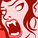# QlikView App Dev

Discussion Board for collaboration related to QlikView App Development.

Announcements
Skip the ticket, Chat with Qlik Support instead for instant assistance.
cancel
Showing results for
Did you mean:Creator

## Convert If condition into Set analysis expression

Can we convert below if condition to a set analysis expression to make it work. Please ,help me with suggestions.

Count (Distinct
If (
([Initial Receipt Date] >= %CPStartDateRRF AND [Initial Receipt Date] <= %CPEndDateRRF AND RRF_State_RPT_Flag = 1)
OR ([CF Follow-Up Date]>= %CPStartDateRRF AND [CF Follow-Up Date] <= %CPEndDateRRF AND RRF_State_RPT_Flag = 1 AND Significant = 'Yes')
, EVENT_COUNTER)
)

Labels (2)

• ### Layout & Visualizations

2 RepliesSpecialist II

Count (
{<[Initial Receipt Date]={">=\$(%CPStartDateRRF)"},[Initial Receipt Date]={"<=\$(%CPEndDateRRF)"},RRF_State_RPT_Flag={1}>
+
<[CF Follow-Up Date]={">=\$(%CPStartDateRRF)"},[CF Follow-Up Date]={"<=\$(%CPEndDateRRF)"},RRF_State_RPT_Flag={1},Significant={'Yes'}>
}
Distinct EVENT_COUNTER)Contributor

@Anuhyak1 wrote:

Can we convert below if condition to a set analysis expression to make it work. Please ,help me with suggestions.

Count (Distinct   my ford benefits
If (
([Initial Receipt Date] >= %CPStartDateRRF AND [Initial Receipt Date] <= %CPEndDateRRF AND RRF_State_RPT_Flag = 1)
OR ([CF Follow-Up Date]>= %CPStartDateRRF AND [CF Follow-Up Date] <= %CPEndDateRRF AND RRF_State_RPT_Flag = 1 AND Significant = 'Yes')
, EVENT_COUNTER)
)

If you just want to ignore some filters you can use your if expression combined with set analysis to ignoreCommunity Browser